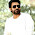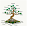» » Method and Type of methods

# Method and Type of methods

### Method:

• Method is a sub block of a class that contains logic of that class.
• logic must be placed inside a method, not directly at class level, if we place logic at class level compiler throws an error.
• So class level we are allowed to place variables and methods.
• The logical statements such as method calls, calculations and printing related statements must be placed inside method, because these statements are considered as logic.

1. package com.instanceofjava;
2. class sample{
3.
4. int a;
5. int b;
6.
7. System.out.println("instance of java"); // compiler throws an error.
8.
9. }
10.

1. package com.instanceofjava;
2. class sample{
3.
4. static int a=10;
5.
6. public static void main(String args[]){

7. System.out.println(a); // works fine,prints a value:10
8.
9.  }
10. }

### Method Terminology:

#### 1.Method Prototype:

• The head portion of the method is called method prototype.
1. package com.instanceofjava;
2. class sample{
3.
4. static int b=10;
5.
6. public static void main(String args[]) // ->method prototype.
7. {

8. System.out.println(b);
9.
10.  }

#### 2.Method body and logic:

• The "{ }" region is called method body, and the statements placed inside method body is called logic.

1. package com.instanceofjava;
2. class sample{

3. public static void main(String args[]) // ->method prototype.
4.
5.   int a=10,b=20; // method logic
6.   int c=a+b;  // method logic
7.   System.out.println(c);  // method logic
8.
9.  }
10. }

#### 3.Method parameters and arguments:

• The variables declared in method parenthesis "( )" are called parameters.
• We can define method with 0 to n number of parameters.
• The values passing to those parameters are called arguments.
• In method invocation we must pass arguments according to the parameters order and type.

1. package com.instanceofjava;
2. class sample{

3. public void add(int a, int b) // ->method prototype. int a, int b are parameters
4.
5.   int c=a+b;  // method logic
6.   System.out.println(c);  // method logic
7.
8.  }
9.
10. public static void main(String args[]) // ->method prototype.
11.
12.   sample obj= new sample();
13.
14.  obj.add(1,2); //method call , here 1, 2 are arguments.

15. }
16. }

#### 4:Method signature:

• The combination of method "name + parameters "  is called method signature

1. package com.instanceofjava;
2. class sample{

3. public void add(int a, int b) // ->method prototype. int a, int b are parameters
4.
5.   int c=a+b;  // method logic
6.   System.out.println(c);  // method logic
7.
8.  }
9.
10. public static void main(String args[]) // ->method prototype.
11.
12.   sample obj= new sample();
13.
14.  obj.add(1,2); //method call , here 1, 2 are arguments.

15. }
16. }

• In the above program add(int a, int b) and  main(String args[]) are method signatures.

#### 5. Method return type:

• The keyword that is placed before method name is called method return type.
• It tells to compiler and JVM about the type of the value is returned from this method after its execution
• If nothing is return by method then we can use "void" keyword which specifies method returns nothing.

1. package com.instanceofjava;
2. class sample{

3. public int add(int a, int b) // ->method prototype. int a, int b are parameters
4.
5.   int c=a+b;  // method logic
6.   return c;
7.  }
8.
9. public static void main(String args[]) // ->method prototype.
10.
11.   sample obj= new sample();
12.
13. int x= obj.add(1,2); //method call , here 1, 2 are arguments.
14. System.out.println(x);

15. }
16. }

#### 1.Method with out return type  and without arguments.

1. package com.instanceofjava;
2. class sample{
3.
5.
6. int a=10;
7. int b=10;
8. int c=a+b;
9. System.out.println(c);
10.
11. }

12. public static void main(String args[]) // ->method prototype.
13.
14. sample obj= new sample();
16.
17.
18.  }
19. }

#### 2.Method with out return type and with arguments.

1. package com.instanceofjava;
2. class sample{
3.
4. public void add(int a, int b){
5.
6. int c=a+b;
7. System.out.println(c);
8.
9. }

10. public static void main(String args[]) // ->method prototype.
11.
12. sample obj= new sample();
14.
15.
16.  }
17. }

#### 3.Method with return type  and without arguments.

1. package com.instanceofjava;
2. class sample{
3.
5. int a=20;
6. int b=30;
7. int c=a+b;
8. return c;
9. }

10. public static void main(String args[]) // ->method prototype.
11.
12. sample obj= new sample();
14. System.out.println(x);
15.
16.  }
17. }

#### 4.Method with return type and with arguments.

1. package com.instanceofjava;
2. class sample{
3.
4. public int add(int a, int b){
5.
6. int c=a+b;
7. return c;
8. }

9. public static void main(String args[]) // ->method prototype.
10.
11. sample obj= new sample();
12.
14. System.out.println(x);
15.
16.  }
17. }

#### Method terminology : main method

• Lets take main method and identify method parts

#### 1.Method Creation with body:

• The process of creating method with body is called method definition.
• Technically this method called concrete method.

1. class A{
2.
4.
5. }
6.
7. }

#### 2.Method Creation without body:

• Creating a method without body is called  "Declaring a method" or "Method declaration".
• Technically this method called "abstract method".
• In method declaration the modifier "abstract" is mandatory and also should be terminated with ";".
• Example : public void add();
• And only abstract class can have abstract method. (in interface we can use , by default all methods are abstract  )

1. abstract class A{
2.
4.
5. }

### Static and non static methods:

• If a method has static keyword in its definition then that method called static method.
• We can call static method directly from main method.
• If method does not have static keyword in its definition then it is a non static method.
• If we want to call a non static method from main method we need object of that class
• if we want to call static method outside the class we can call by using classname.method();

1. class A{
2.
3. public static void show(){    // static method
4.  System.out.println("static method");
5.
6. public void display(){      //non static method
7.  System.out.println("non static method");
8.
9. public static void main(String[] args){
10. show(); // static method call
11. A obj= new A();
12. obj.display(); // non static method call
13. }
14. }

#### Instance Of Java

1.2.